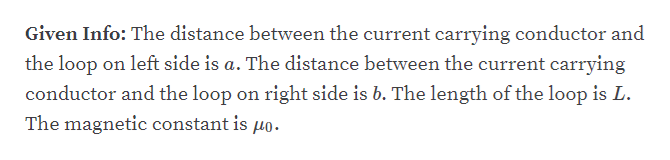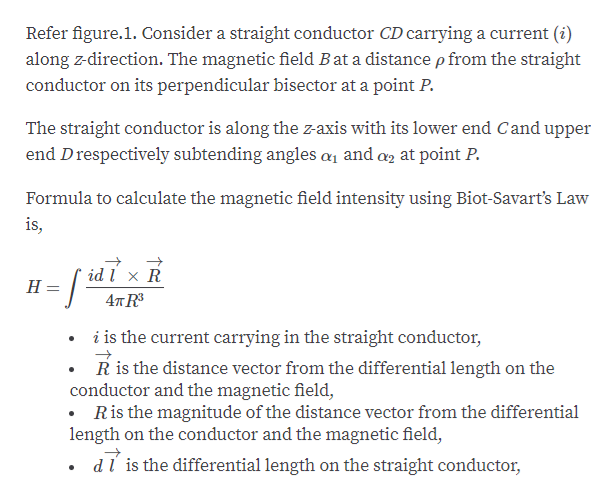# Figure E29.7В- draA

Question
72 views

29.7       The current in the long, straight wire AB shown in Fig. E29.7 is upward and is increasing steadily at a rate di/dt.

(a) At an instant when the current is i, what are the magnitude and direction of the field B at a distance  r to the right of the wire?

(b) What is the magnetic flux through the narrow, shaded strip?

(c) What is the total flux through the loop?

(d) What is the induced emf in the loop?

(e) Evaluate the numerical value of the induced emf if a = 12.0 cm, b = 36.0 cm, L = 24.0 cm, and di/dt = 9.60 A/s.

check_circle

Step 1help_outlineImage TranscriptioncloseGiven Info: The distance between the current carrying conductor and the loop on left side is a. The distance between the current carrying conductor and the loop on right side is b. The length of the loop is L The magnetic constant is uo. fullscreen
Step 2
Step 3help_outlineImage TranscriptioncloseRefer figure.1. Consider a straight conductor CD carrying a current (i) along z-direction. The magnetic field Bat a distance p from the straight conductor on its perpendicular bisector at a point P The straight conductor is along the zaxis with its lower end Cand upper end Drespectively subtending angles a1 and a at point P. Formula to calculate the magnetic field intensity using Biot-Savart's Law is, id l x R Н. 4т R i is the current carrying in the straight conductor, R is the distance vector from the differential length on the conductor and the magnetic field, .Ris the magnitude of the distance vector from the differential length on the conductor and the magnetic field, dl is the differential length on the straight conductor fullscreen

### Want to see the full answer?

See Solution

#### Want to see this answer and more?

Solutions are written by subject experts who are available 24/7. Questions are typically answered within 1 hour.*

See Solution
*Response times may vary by subject and question.
Tagged in

### Current Electricity